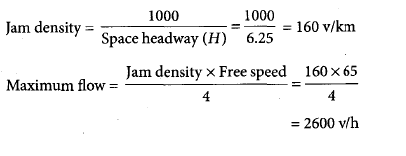Courses

# Test: Transportation Engineering- 2

## 20 Questions MCQ Test GATE Civil Engineering (CE) 2022 Mock Test Series | Test: Transportation Engineering- 2

Description
This mock test of Test: Transportation Engineering- 2 for GATE helps you for every GATE entrance exam. This contains 20 Multiple Choice Questions for GATE Test: Transportation Engineering- 2 (mcq) to study with solutions a complete question bank. The solved questions answers in this Test: Transportation Engineering- 2 quiz give you a good mix of easy questions and tough questions. GATE students definitely take this Test: Transportation Engineering- 2 exercise for a better result in the exam. You can find other Test: Transportation Engineering- 2 extra questions, long questions & short questions for GATE on EduRev as well by searching above.
QUESTION: 1

### For carrying out bituminous patch work during the rainy season, the most suitable binder is

Solution:

Emulsion is generally used for cold and wet climate condition. For bituminous patch work during rainy season bituminous emulsion is used.

QUESTION: 2

### Bituminous materials are commonly used in highway construction because of their good

Solution:

Bitumen is derived from fraction distillation of petroleum and is commonly used because of binding and water-proofing properties. These properties prevent water going down to subgrade and thus, leading to better strength of the flexible pavement.

QUESTION: 3

### In cement concrete pavements, tie bars are installed in

Solution:
QUESTION: 4

The following general statement may be made about the penetration value and softening point of bitumen

Solution:

Penetration Test- Represents hardness/softness of bitumen by measuring the depth of penetration 1/10 of mm to which a standard needle carrying a weight of 100 gram penetrates in 5 seconds.
Softening point Test- The temperature at which a standard ball passes through the sample of bitumen and falls when heated under water or glycerine. It represents the fluidity of binder.
Higher the penetration value means the needle will go into larger depth while performing penetration test. Thus, high penetration generally means the consistency of bitumen is soft and thus, can be turned into liquid even at low temperatures. This results in lower softening point.

QUESTION: 5

The minimum length of the overtaking zone should be

Solution:
QUESTION: 6

In case of the valley curves or sag curves the maximum possible deviation angle is obtained when

Solution: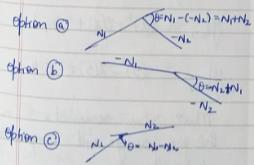QUESTION: 7

The bitumen with viscosity reduced by volatile diluents is known as

Solution:

Bitumen Emulsion- Bitumen is dispersed in fine globules in water.
Cutback Emulsion- Volatile dilutants makes the bitumen easy to be laid. Once laid easily the dilutant evaporates due to its volatile nature.
Asphalt- Also known as Bitumen is a sticky, black and highly viscous liquid or semi-solid form of petroleum.

QUESTION: 8

On hill roads, the necessity to ease the gradient at horizontal curves by an amount to offset the extra tractive effort involved at curves is known as

Solution:
QUESTION: 9

According to IRC guidelines, the maximum volume of traffic that a rotary can efficiently handle is

Solution:
QUESTION: 10

The maximum number of passenger cars that can pass a given point on a lane or roadway during one hour under ideal roadway and traffic conditions is known as

Solution:
QUESTION: 11

For a highway with the design speed of 50 km/h, coefficient of friction 0.5, perception-brake reaction time of 2.5 s, and average vehicle length 5 m, the spacing between the vehicle length in their movement will be

Solution: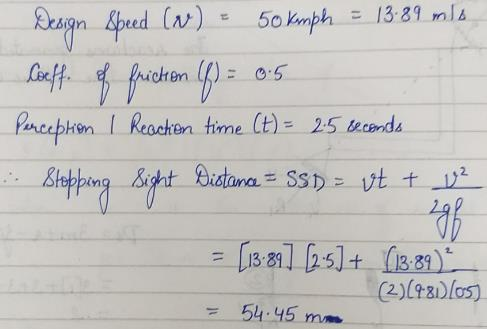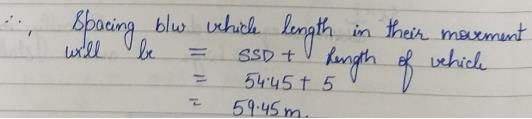QUESTION: 12

For a two-lane pavement with radius of curve of 100 m, with design speed of 65 km/h & wheelbase of design vehicle of 6 m, the extra widening necessary will be

Solution: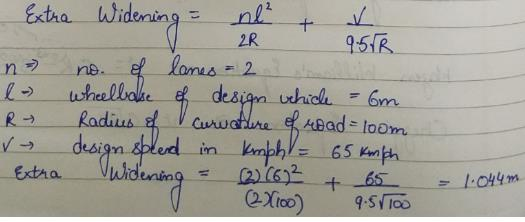QUESTION: 13

Consider the following factors:

1. Reaction time    2. Speed

3. Coefficient of the longitudinal friction

Which of these factors are taken into account for computing braking distance?

Solution:
QUESTION: 14

Match List-I with List-II and select the correct answer using the codes given below the lists: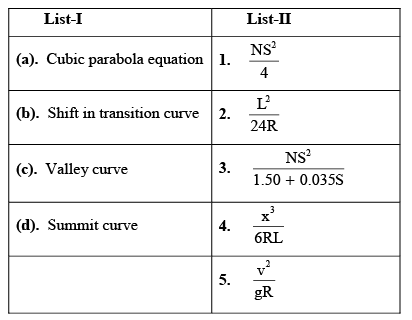Solution:
QUESTION: 15

Which one of the following represents the basic capacity of a single lane road? Where, V = speed in km/h, s = average centre to centre spacing of vehicle in m, and h = average time headway between two vehicle in seconds

Solution:
QUESTION: 16

Which one of the following method of O-D traffic surveys is conducted for comprehensive analysis of traffic and transportation data?

Solution:
QUESTION: 17

Consider the following parameters related to a rotary intersection:

1. Width of the weaving section
2. Length of the weaving section
3. Proportion of weaving traffic
4. Weaving angle
5. Width of the carriageway at entry

Capacity is generally expressed in terms of

Solution:
QUESTION: 18

If the normal flows on two approach roads at an intersection are respectively 500 PCU/h and 300 PCU/h, the saturation flows are 1600 PCU/h and 300 CPU/h, the saturation flows are 1600 PCU/h on each road and the total lost time per single cycle is 16 s, then the optimum cycle time by Webster’s method is

Solution: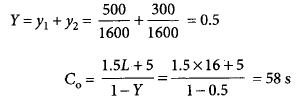QUESTION: 19

When two roads with two-lane, two-way traffic, cross at an uncontrolled intersection, the total number of potential major conflict points would be __________.

Solution:
QUESTION: 20

On a road the free speed was 65 km/h and the space headway at jam density was 6.25 vpkm. What is the maximum flow which could be expected on this road?

Solution: